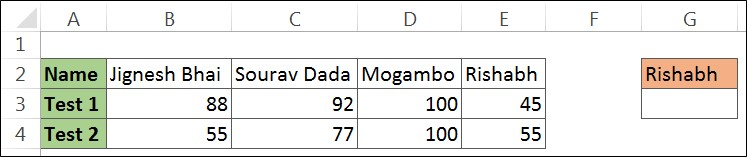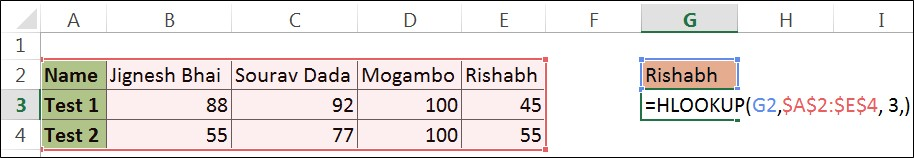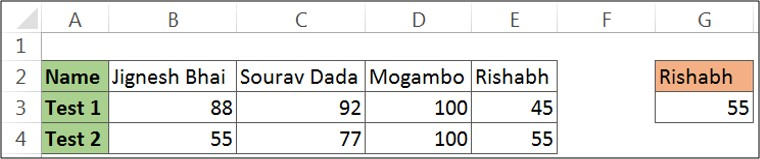# How to use Excel Hlookup FormulaHlookup Formula and Vlookup Formula together in Excel can be called siblings. Vlookup stands for Vertically Viewing in Formula, similarly as Hlookup stands for Horizontally Viewing in Formula. Most data are available in Vertical Format, so the use of Hlookup Formula in Excel is greatly reduced.

Hlookup Formula is available in all Excel Version 2007 – 2016. Like Vlookup Formula, Hlookup Formula also helps in finding Exact Match, approximate match, and wildcard characters. Hlookup Formula is categorized under Lookup / Reference. Hlookup is an in-built task in Excel. It is a worksheet function and can be entered as part of Excel Formulas.50% OFF on Excel Course

• Get 2-Year Access with unlimited views of 220+ videos
• Use Coupon code - NAV50OFF & Start learning

Hlookup is defined as “a function that looks for the value in the first row of data and returns the value of the same column from the specified row”.

Syntax of Hlookup Function:

The syntax of the HLOOKUP function is similar to that of the VLOOKUP function. The only difference is that in Vlookup we used to search for columns but in Hlookup Formula we would search for the row.

Where,

LOOKUP_VALUE: It is the parameter that is most important. This includes the value we are actually looking for. In other words, this is the value that we have to lookup to.

TABLE_ARRAY: The second parameter is also an important parameter. This provides the range in which our lookup value and result value are located. However, it should be noted that we have to select the data in such a way that our lookup value should be from the first row of the selection range.

ROW_INDEX NUMBER: The third parameter is the answer we are looking for. Or we can say that this is the row number from which you want to retrieve your data. The row_index number must not exceed the selected range.

RANGE_LOOKUP: The fourth parameter is optional. In this parameter, we decide whether to find an exact match of the value given in the first parameter or an approximate match. If you do not enter anything in this argument, it will select the default option TRUE. TRUE means an approximate match.

Example of Hlookup Formula in Excel:

Suppose we have some names of students with scores scored in two texts. The objective of this example is to find the marks scored by Rishabh in Test 2. To achieve this objective follow these steps –

Step 1: In our example, we have entered Rishabh as Lookup Value in cell G2.Step 2: Start entering arguments in Hlookup Formula:

a. LOOKUP_VALUE: We want to find the marks of Rishabh in Test2. So our lookup_value will be H2

b. TABLE_ARRAY: In this argument, enter the table reference as \$ A \$ 2: \$ E \$ 4.

c. ROW_INDEX NUMBER: Enter the row number from where we want to get the value. Here we will enter 3 Because Test2 is in the third row of our table selection.

d. RANGE_LOOKUP: Enter False here as we want to match Exact Match to Match.The Hlookup Formula will be =HLOOKUP(H2,\$A\$2:\$E\$4,3,FALSE)

Step 3: Press EnterPoints to Remember:

1. Hlookup Formula is not a sensitive matter. It does not differentiate between uppercase and lowercase
2. The Lookup value must be on the topmost row of the Table Array.
3. If we leave to enter the range lookup then it will use the default option ie TRUE.
4. If Hlookup Exact cannot find a match it will return a value less than the Lookup value.
5. Hlookup Formula similarly supports wildcard characters in the lookup argument as done by VLOOKUP.
6. If the range Lookup is False and the Lookup value is not found in the defined table array, it will return # N / A Error.
7. If we enter a row index number less than 1, it will be #VALUE! The error will return. And if the row index number is greater, then it’s #REF! The error will return.
8. Table_array must be sorted in ascending order.
9. The range_Lookup argument needs to be set to FALSE when you want wildcard characters to function correctly.

So, it was about the Hlookup Formula in Excel. How to use Hlookup Formula and what points to keep in mind when working with Hlookup Formula in Excel.